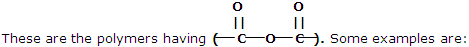chem

Explain how dissolving the Group IV carbonate precipitate with 6M CH3COOH, followed by the addition of extra acetic acid.

#### Related Questions in Chemistry

• ##### Q :Define alum Illustrate alum?

Illustrate alum?

• ##### Q :Calculate molarity of a solution

Provide solution of this question. Molarity of a solution prepared by dissolving 75.5 g of pure KOH in 540 ml solution is: (a) 3.05 M (b) 1.35 M (c) 2.50 M (d) 4.50 M

• ##### Q :Mole fraction Give me answer of

Give me answer of following question. The sum of the mole fraction of the components of a solution is : (a) 0 (b) 1 (c) 2 (d) 4.

• ##### Q :What is Spectroscopy? This is a very

This is a very important aspect of Physical Chemistry in which knowledge of the size, shape, rigidity and electronic structure of molecules deduced from the experimental methods treated here goes hand in hand with the theoretical approaches of chemical reactions. Spec

• ##### Q :P block bif3 is ionic while other

bif3 is ionic while other trihalides are covalent in nature

• ##### Q :Atmospheric pressure Give me answer of

Give me answer of this question. The atmospheric pressure is sum of the: (a) Pressure of the biomolecules (b) Vapour pressure of atmospheric constituents (c) Vapour pressure of chemicals and vapour pressure of volatile (d) Pressure created on to atmospheric molecules

• ##### Q :Precipitation Addition of conc. HCl to

Addition of conc. HCl to saturated Bacl2 solution precipitates Bacl2 ; because of the following reason : (a) It follows from Le Chatelier's principle (b) Of common-ion effect (c) Ionic product (Ba++)(cl) remains constant in a saturated sol

• ##### Q :What are condensation polymers? Give

These types of polymers are formed as a result of condensation reaction between monomer units. Some common examples are being discussed here:

1. Polyesters##### Q :Why acetic has less conductivity than

Illustrate the reason, why acetic has less conductivity than Hcl?

• ##### Q :Problem based on molecular weight

Select the right answer of the question. Molecular weight of urea is 60. A solution of urea containing 6g urea in one litre is : (a)1 molar (b)1.5 molar (c) 0.1 molar (d) 0.01 molar